# How to count characters in Excel: total or only specific characters in a cell / range

The tutorial explains how to count characters in Excel. You will learn the formulas to get the total character count in a range, and count only specific characters in a cell or in several cells.

Our previous tutorial introduced the Excel LEN function, which allows counting the total number of characters in a cell.

The LEN formula is useful on its own, but in liaison with other functions such as SUM, SUMPRODUCT and SUBSTITUTE, it can handle far more complex tasks. Further on in this tutorial, we are going to have a closer look at a few basic and advanced formulas to count characters in Excel.

## How to count all characters in a range

When it comes to counting a total number of characters in several cells, an immediate solution that comes to mind is to get the character count for each cell, and then add up those numbers:

`=LEN(A2)+LEN(A3)+LEN(A4)`

Or

`=SUM(LEN(A2),LEN(A3),LEN(A4))`

The above formulas might work fine for a small range. To count total characters in a bigger range, we'd better come up with something more compact, e.g. the SUMPRODUCT function, which multiplies the arrays and returns the sum of the products.

Here's the generic Excel formula to count characters in a range:

=SUMPRODUCT(LEN(range))

And your real-life formula may look similar to this:

`=SUMPRODUCT(LEN(A1:A7))`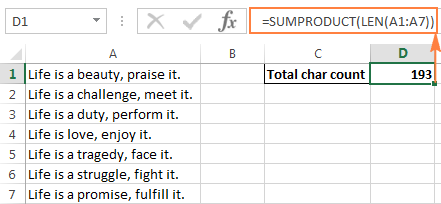Another way to count characters in a range is to use the LEN function in combination with SUM:

`=SUM(LEN(A1:A7))`

Unlike SUMPRODUCT, the SUM function does not calculate arrays by default, and you need to press Ctrl + Shift + Enter to turn it into an array formula.

As demonstrated in the following screenshot, the SUM formula returns the same total character count: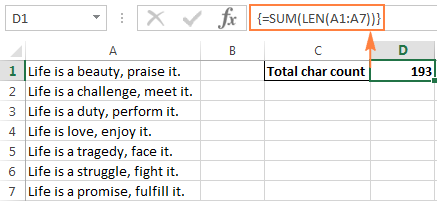#### How this range character count formula works

This is one of the most straightforward formulas to count characters in Excel. The LEN function calculates the string length for each cell in the specified range and returns them as an array of numbers. And then, SUMPRODUCT or SUM adds up those numbers and returns the total character count.

In the above example, an array of 7 numbers that represent the lengths of strings in cells A1 to A7 is summed: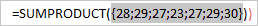Note. Please pay attention that the Excel LEN function counts absolutely all characters in each cell, including letters, numbers, punctuation marks, special symbols, and all spaces (leading, trailing and spaces between words).

## How to count specific characters in a cell

Sometimes, instead of counting all characters within a cell, you may need to count only the occurrences of a specific letter, number, or special symbol.

To count the number of times a given character appears in a cell, use the LEN function together with SUBSTITUTE:

=LEN(cell)-LEN(SUBSTITUTE(cell, character,""))

To better understand the formula, consider the following example.

Suppose, you maintain a database of delivered items, where each item type has its own unique identifier. And each cell contains several items separated by comma, space, or any other delimiter. The task is to count how many times a given unique identifier appears in each cell.

Assuming that the list of delivered items is in column B (beginning in B2), and we are counting the number of "A" occurrences, the formula is as follows:

`=LEN(B2)-LEN(SUBSTITUTE(B2,"A",""))`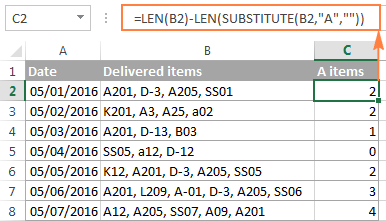#### How this Excel character count formula works

To understand the formula's logic, let's break it down into smaller parts:

• First, you count the total string length in B2:
`LEN(B2)`
• Then, you use the SUBSTITUTE function to remove all occurrences of letter "A" in B2 by replacing it with an empty string (""):
`SUBSTITUTE(B2,"A","")`
• And then, you count the string length without "A" character:
`LEN(SUBSTITUTE(B2,"A",""))`
• Finally, you subtract the length of the string without "A" from the total length string.

As the result, you get the count of "removed" characters, which equals to a total number of that character occurrences in the cell.

Instead of specifying the character you want to count in a formula, you can type it in some cell, and then reference that cell in a formula. In this way, your users will be able to count occurrences of any other character they input in that cell without tampering with your formula: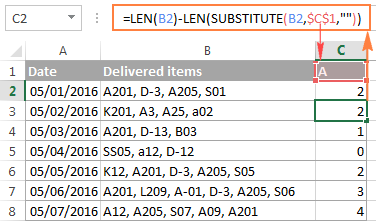Note. Excel's SUBSTITUTE is a case-sensitive function, and therefore the above formula is case-sensitive as well. For example, in the above screenshot, cell B3 contains 3 occurrences of "A" - two in uppercase, and one in lowercase. The formula has counted only the uppercase characters because we supplied "A" to the SUBSTITUTE function.

### Case-insensitive Excel formula to count specific characters in a cell

If you need a case-insensitive character count, embed the UPPER function inside SUBSTITUTE to convert the specified character to uppercase before running the substitution. And, be sure to enter the uppercase character in the formula.

For example, to count "A" and "a" items in cell B2, use this formula:

`=LEN(B2)-LEN(SUBSTITUTE(UPPER(B2),"A",""))`

Another way is to use nested Substitute functions:

`=LEN(B2)-LEN(SUBSTITUTE(SUBSTITUTE (B2,"A",""),"a","")`

As you can see in the below screenshot, both formulas flawlessly count uppercase and lower case occurrences of the specified character: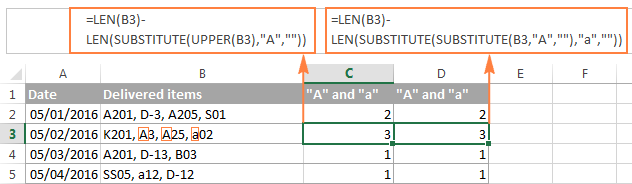In some cases, you may need to count many different characters in a table, but you may not want to modify the formula each time. In this case, nest one Substitute function within another, type the character you want to count in some cell (D1 in this example), and convert that cell's value to uppercase and lowercase by using the UPPER and LOWER functions:

`=LEN(B2)-LEN(SUBSTITUTE(SUBSTITUTE(B2, UPPER(\$D\$1), ""), LOWER(\$D\$1),""))`

Alternatively, convert both the source cell and the cell containing the character either to uppercase or lowercase. For example:

`=LEN(B2)-LEN(SUBSTITUTE(UPPER(B2), UPPER(\$C\$1),""))`

The advantage of this approach is that regardless of whether the uppercase or lowercase character is input in the referenced cell, your case-insensitive character count formula will return the right count: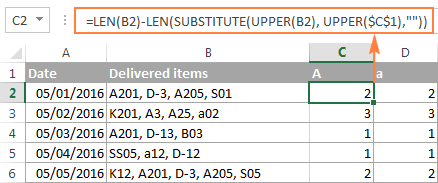### Count occurrences of certain text or substring in a cell

If you want to count how many times a specific combination of characters (i.e. certain text, or substring) appears in a given cell, e.g. "A2" or "SS", then divide the number of characters returned by the above formulas by the length of the substring.

Case-sensitive formula:

`=(LEN(B2)-LEN(SUBSTITUTE(B2, \$C\$1,"")))/LEN(\$C\$1)`

Case-insensitive formula:

`=(LEN(B2)-LEN(SUBSTITUTE(LOWER(B2),LOWER(\$C\$1),"")))/LEN(\$C\$1)`

Where B2 is the cell containing the entire text string, and C1 is the text (substring) you want to count.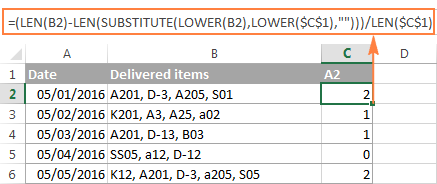For the detailed explanation of the formula, please see How to count specific text / words in a cell.

## How to count specific character(s) in a range

Now that you know an Excel formula to count characters in a cell, you may want to improve it further to find out how many times a certain character appears in a range. For this, we will take the Excel LEN formula to count a specific char in a cell discussed in the previous example, and put it inside the SUMPRODUCT function that can handle arrays:

SUMPRODUCT(LEN(range)-LEN(SUBSTITUTE(range, character,"")))

In this example, the formula takes the following shape:

`=SUMPRODUCT(LEN(B2:B8)-LEN(SUBSTITUTE(B2:B8, "A","")))`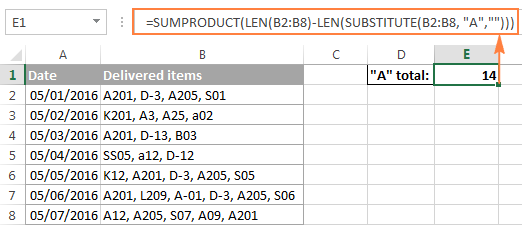And here's another formula to count characters in Excel's range:

`=SUM(LEN(B2:B8)-LEN(SUBSTITUTE(B2:B8, "A","")))`

Compared to the first formula, the most obvious difference is using SUM instead of SUMPRODUCT. Another difference is that it requires pressing Ctrl + Shift + Enter because unlike SUMPRODUCT, which is designed to process arrays, SUM can handle arrays only when used in an array formula.

If you don't want to hardcode the character in the formula, you can of course type it in some cell, say D1, and reference that cell in your character count formula:

`=SUMPRODUCT(LEN(B2:B8)-LEN(SUBSTITUTE(B2:B8, D1,"")))`

Note. In situations when you count the occurrences of a specific substring in a range (e.g. orders beginning with "KK" or "AA"), you need to divide the character count by the substring length, otherwise each character in the substring will be counted individually. For example:

`=SUM((LEN(B2:B8)-LEN(SUBSTITUTE(B2:B8, D1, ""))) / LEN(D1))`

#### How this character counting formula works

As you may remember, the SUBSTITUTE function is used to replace all occurrences of the specified character ("A" in this example) with an empty text string ("").

Then, we supply the text string returned by SUBSTITUTE to the Excel LEN function so that it calculates the string length without A's. And then, we subtract that character count from the total length of the text string. The result of these calculations is an array of character counts, with one character count per cell.

Finally, SUMPRODUCT sums the numbers in the array and returns the total count of the specified character in the range.

### A case-insensitive formula to count specific characters in a range

You already know that SUBSTITUTE is a case-sensitive function, which makes our Excel formula for character count case-sensitive as well.

To make the formula ignore case, follow the approaches demonstrated in the previous example: Case-insensitive formula to count specific characters in a cell.

In particular, you can use one of the following formulas to count specific characters in a range ignoring case:

• Use the UPPER function and enter a character in uppercase:

`=SUMPRODUCT(LEN(B2:B8) - LEN(SUBSTITUTE(UPPER(B2:B8),"A","")))`

• Use nested SUBSTITUTE functions:

`=SUMPRODUCT(LEN(B2:B8) - LEN(SUBSTITUTE(SUBSTITUTE((B2:B8),"A",""),"a","")))`

• Use UPPER and LOWER functions, type either an uppercase or lowercase char in some cell, and reference that cell in your formula:

`=SUMPRODUCT(LEN(B2:B8) - LEN(SUBSTITUTE(SUBSTITUTE((B2:B8), UPPER(\$E\$1), ""), LOWER(\$E\$1),"")))`

The below screenshot demonstrates the last formula in action: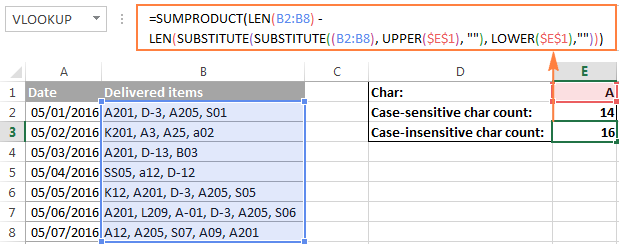Tip. To count the occurrences of a specific text (substring) in a range, use the formula demonstrated in How to count specific text / words in a range.

This is how you can count characters in Excel using the LEN function. If you want to know how to count words rather than individual characters, you will find a few useful formulas in our next article, please stay tuned!

In the meantime, you can download a sample workbooks with character count formula discussed in this tutorial, and check out a list of related resources at the end of the page. I thank you for reading and hope to see you soon!

## You may also be interested in

### 48 comments to "How to count characters in Excel: total or only specific characters in a cell / range"

1.Rick says:

I have a list of names.

e.g.
Bob Anderson
Alexander Trifuntov
John Andrus
Andre Johnson

I want a formula to tell me what the most common string of 3 letters is?

If it involves VB that is fine. It is the logic of the coding that I haven't been able to create. I can just use CTRL+F to put in the ones I THINK are most common, but I might be missing something. And there are 3018 names in my list.

In this case we know, by brute force, observation that it is "a-n-d". Answer: 4 times it happens in this list. The spreadsheet is uses Tab and space to create the delimiters. So, separate cell for each part of the names.

Thank You.

•Rick says:

Hey,

You have taught me well. I got it:

{=INDEX(range,MODE(XMATCH(range,range,0)))} *({} Array brackets from CTRL SHIFT ENTER)

I would still like to know a better way. :)

•Rick says:

Sorry. It worked to tell me most common item in list but I still need to know how to get most common "piece" of the items. ;) Sigh.

2.Dhiraj says:

I want to count character between 2 rows at a time, where change in char. took place; :
ex.
1) abcd-.123.456.77
2) abcd-.123.456.12345
Like in above ex. Changes taken after 14 charecter.,
So Ans. Should be 14, it should be automated by applying just formula, instead of count manually.
Thanks.

•Alexander Trifuntov (Ablebits Team) says:

Hello!
The formula below will do the trick for you:

=MATCH(FALSE,MID(A1,ROW(1:99),1)=MID(A2,ROW(1:99),1),0)

3.Ramraj says:

Hi
Can anyone help me with a formula.
I want to count the numbers in a range ( For this I can use countif ), but I want to set max limit.
Ex: IF A1 to Z1 is the range in which I want to count number of "B". But I want to set limit of 10. If B count is more than 10, then my result should be 10 & If B count is 6, then my result should be 6.

•Alexander Trifuntov (Ablebits Team) says:

Hi,
Please check the formula below, it should work for you:

=IF(COUNTIF(D2:D20,5)>10,10,COUNTIF(D2:D20,5))

4.pritesh says:

hi sir
pls give a Autogenerate serial number formula.

for example
column a column B
receipt REC-001
PAYMENTS PAY-001
RECEIPT REC-002
RECEIPT REC-003
PAYMENTS REC- 002

5.Hasan says:

Hello everyone,
can anyone help me please to do the same above process for counting words in mixed cell?

•Alexander Trifuntov (Ablebits Team) says:

Hello!
I’m sorry but your task is not entirely clear to me. For me to be able to help you better, please describe your task in more detail. Please specify what you were trying to find, what formula you used and what problem or error occurred. Give an example of the source data and the expected result.
It’ll help me understand it better and find a solution for you.

6.D Krishna Kumar says:

a1 Cell is containing following text -
"'1.5+2.6+3+2.4+0.5"
I want following result B1 cell ( Sum total of the A1) "=1.5+2.6+3+2.4+0.5" (which is displayed as 10)
Basically I want to change the text string to a formula
Thanks

•Alexander Trifuntov (Ablebits Team) says:

Hello!
You can only convert text to an Excel formula using a VBA macro.

•D Krishna Kumar says:

Can you share the VBA Code for that. Whenever I try it in (my limited knowledge of VBA), it goes back to the same text string.
Thanks

•Alexander Trifuntov (Ablebits Team) says:

Hello!

7.Goenie says:

Hi, I'm trying to string together a formula,but not yet quite succeeding.
Case: We have 100 production orders. on these orders are individual materials. One specific material can be on a production order multiple times.
I want to count on how many production orders a specific material is used, not counting the double entries of a material on the same production order.
Example:
- Order A > material 1, 2, 3, 2, 1, 6
- Order B > material 1, 2, 4, 5, 5
Required outcome:
- material 1 on 2 orders = count is 2
- material 2 on 2 orders (1 double entry) = count is 2
- material 3 on 1 order = count is 1
- material 5 on 1 order (1 double entry) = count is 1
etc

GREATLY appreciated! :-)
John

•Alexander Trifuntov (Ablebits Team) says:

Hello!
The information presented to you is not enough to give you advice. How many cells does the text "Order A> material 1, 2, 3, 2, 1, 6" take? One? Two? Seven? Write an example of the source data and the result you want to get. Please describe your problem in more detail. It’ll help me understand it better and find a solution for you. Thank you.

8.GORDHAN LAL KUMAWAT says:

a1 Cell is containing following formula -
=53.95+56.26+90
I want following result B1 cell ( how many values have been added or how many "+" sign)
result = 3

•Alexander Trifuntov (Ablebits Team) says:

Hello
If I understand your task correctly, the following formula should work for you:

=LEN(FORMULATEXT(A1)) - LEN(SUBSTITUTE(FORMULATEXT(A1),"+","")) + 1

9.Vishal says:

If I need to start count from right end of the cell, instead of left, how do we do that?

•Alexander Trifuntov (Ablebits Team) says:

Hello Vishal!
To determine the position number counting from the right, from the total number of characters (LEN function), subtract the position number counting from the left (FIND function or SEARCH)

10.kit kerner says:

Building contractors must have a 'tool' that can tell them how many distinct numbers then need to place a 'unit' number on a dwelling. For example a builder is building a place that has 144 units and they want to place a unit number on the door (or somewhere). When the numbers are purchased, how many ones, or twos or fours are required to get the job done, without 'writing' it out?

11.Robert Cox says:

I want to count the number of times the letter “D” is used in a row. Columns C to G with the total in column I. Can you please help.

•Alexander Trifuntov (Ablebits Team) says:

Hello Robert!
If I understand your task correctly, maybe the following formula should work for you:

=SUM(LEN(C1:G1) - LEN(SUBSTITUTE(C1:G1,"D","")))

12.K Krishnamurthy says:

Hi
This is a attendance sheet in excel. What is the formula to get a count of only P in total column.
Sl.no. 1st April 2nd April 3rd April 4th April 5th April 6th April Total
1 P P P P P W/O ?

•Alexander Trifuntov (Ablebits Team) says:

Hello!
If each character is typed in a separate cell, you can use the following formula to count the total number of "P":

=COUNTIF(A9:H9,"P")

If all the characters are in one cell, then please try out this formula:

=LEN(A2)-LEN(SUBSTITUTE(A2,"P",""))

Hope you’ll find this information helpful.

13.Rajni Bala says:

i want count only character not space ,special symble Please solve my problem

14.Rakesh Verma says:

How can I count a text "H" in different mixed columns Alphabatic and numeric

15.Devis says:

Hello everyone
i am trying to use this formula to count how many "m" characters are in specific cells in different sheets {FORMULA =SUMPRODUCT(LEN(Histori!H3+Matematike!L3+Fiskulture!K3+'B. sipermarrjes'!K3+Mjedis!H3)-LEN(SUBSTITUTE(Histori!H3+Matematike!L3+Fiskulture!K3+'B. sipermarrjes'!K3+Mjedis!H3,"m","")))} but every time it finds the character i get #VALUE!.
Can anyone help me?

16.RANJAN KUMAR RAKESH says:

How can I count tom occurences in given single cells exp like Tom,Raj,tom,tom with the help of sumproduct and Len formula please help me for this

17.Navnath Shinde says:

I want formula to remove right side alpha character till number number.
for example number is:- MH15HY5859AAAA then result should be - MH15HY5859
MH15HY5859A then result should be - MH15HY5859

18.Jeyner Lopez says:

Hi, Sorry if I am missing something, but I didn't see a case when you need count times certain number happen in a sequence, example I have a column A2:A2700 with a random list, I would like to count how many time 2 is after 1, and the same for each numbers, the numbers are from 1 to 48 in a random sequence.

19.Doug says:

Kino:
What do you mean by "same digit on the right of the cell"?

20.kino says:

example 1005000055555
How to count same digit on the right of the cell

•RakeshD says:

=LEN(A1)-LEN(SUBSTITUTE(A1,A2,""))
Put value in "A1" cell & Enter your lookup Value in "A2"
i.e. A1 = 1005000055555, A2 = 0 then output would be "6"

21.Same says:

i am looking for a formula to count the following continous numbers in different cells 111111011101111. I need the answer to be four i.e. only the last four characters to be counted. Any assistance would be appreciated .

22.Eyon says:

This is perfect!

How to count specific character(s) in a range
=SUMPRODUCT(LEN(range)-LEN(SUBSTITUTE(range, character,"")))
=SUMPRODUCT(LEN(B2:B8)-LEN(SUBSTITUTE(B2:B8, "A","")))

I've been trying to find a solution like this for years.

Thank you so much!

23.Tom Hua says:

How can I make the character appears higher than other characters in the same cell. For example:
12th of January (the twelfths day of Jan)
X(2X+2) (X to the power of 2X+2)

24.Ray says:

For your first example of an array formula, using SUM and LEN, I think you inadvertently reprinted the formula from SUMPRODUCT right before. Shouldn't the formula be {=SUM(LEN(A1:A7))}?

25.Ian Galyer says:

Do do i get the text after a number of instances of a unique char
from
E:\Data Bases\Access VB and stuff\Cell references and Date and Time.xlsx

I want to get the text between the 2nd and 3rd "\" and the text after the last "\"
1. Access VB and stuff
2. Cell references and Date and Time.xlsx

Cheers
Ian

•Gennady Terekhov (Ablebits Team) says:

Hello,

I can suggest you the following formulas:

1. =MID(A1,FIND("\",A1, FIND("\",A1)+1)+1,FIND("\",A1,FIND("\",A1,FIND("\",A1, FIND("\",A1)+1))+1)-FIND("\",A1, FIND("\",A1)+1)-1)

2. =RIGHT(A1,LEN(A1)-FIND("\",A1,FIND("\",A1,FIND("\",A1, FIND("\",A1)+1))+1))

where cell A1 is “E:\Data Bases\Access VB and stuff\Cell references and Date and Time.xlsx”

26.Mohanam says:

Hi, How to count the total of a specify letter (exa. N) from efferent columns.

27.Jibann Puri says:

Hello, could you please solve the below issue with excel
i have many more "YES" or "NO" on my Excel Row i wannn to count of how many "YES" or "NO" in the Row Which formula is better on the case.

28.Kalyan says:

Hi, could you please solve the below issue with Macro. I have a Data with different names but not exact match. I want the maxi matched text displayed in other row or column. Match Names1 with Names 2 and seprate the values.

Please past the below names in excel.
Names 1 Names 2
john.nikki 0123-12 jhon-01, nikki
Rosa 8788-a45- Rosa;R, 56 & German
Y.Cristina ycristina.All-654.09, As
ThomasAndrew GR 56-as, Thomas.gr&Andrew.M/for
All are ok 123 All.are.ok,now @ 123
Need solution, for Solution for
examples example.Needsolution

Kalyan Challa

29.ARNAB says:

Hi,
Could you please solve my problem?
I want to count continue a letter.
Here I am trying to explain that, how many "P" is continue to 6 days.
1 2 3 4 5 6 7 8 9 10 11 12 13 14 15 16 17 18 19 20 21 22 23 24 25 26
P P P P P P P P P P P P P P P P P P P P P P P P

Result will be : 3. 3 time its count continue 6P.(1 to 6, 13-18,19-24)

How this is count in excel?if there is any formula,pls help.

30.anand baldha says:

How to count numbers in cell Exp:-6,4,9,10=4

31.sriram pydah says:

How to count specific characters in a range in a specific date as per the above example

32.Vinayaka R Shenoy says:

Hi,
I have a Question,

If i Enter a single word in Cell, Entire Row color Should be change.